Processing ......FreeComputerBooks.com Links to Free Computer, Mathematics, Technical Books all over the World

O'Reilly® Think Stats, 2nd Edition: Exploratory Data Analysis in Python
Top Free Unix/Linux Books 🌠 - 100% Free or Open Source!
• Title: Think Stats, 2nd Edition: Exploratory Data Analysis in Python
• Author(s) Allen B. Downey
• Publisher: O'Reilly Media; 2 edition (November 7, 2014)
• Paperback: 226 pages
• eBook: HTML and PDF (242 pages, 1.8 MB)
• Language: English
• ISBN-10: 1491907339
• ISBN-13: 978-149190733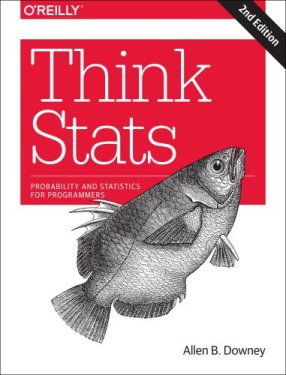Book Description

If you know how to program, you have the skills to turn data into knowledge, using tools of probability and statistics. This concise introduction shows you how to perform statistical analysis computationally, rather than mathematically, with programs written in Python.

You'll work with a case study throughout the book to help you learn the entire data analysis process from collecting data and generating statistics to identifying patterns and testing hypotheses. Along the way, you'll become familiar with distributions, the rules of probability, visualization, and many other tools and concepts.

• Develop your understanding of probability and statistics by writing and testing code
• Run experiments to test statistical behavior, such as generating samples from several distributions
• Use simulations to understand concepts that are hard to grasp mathematically
• Learn topics not usually covered in an introductory course, such as Bayesian estimation
• Import data from almost any source using Python, rather than be limited to data that has been cleaned and formatted for statistics tools
• Allen Downey is a Professor of Computer Science at Olin College of Engineering. He has taught at Wellesley College, Colby College and U.C. Berkeley. He has a Ph.D. in Computer Science from U.C. Berkeley and Master's and Bachelor's degrees from MIT.
Reviews, Rating, and Recommendations: Related Book Categories: Read and Download Links:Similar Books:
•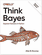O'Reilly® Think Bayes: Bayesian Statistics in Python

If you know how to program, you're ready to tackle Bayesian statistics. With this book, you'll learn how to solve statistical problems with Python code instead of mathematical formulas, using discrete probability distributions rather than continuous mathematics.

•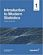Introduction to Modern Statistics (Mine Çetinkaya-Rundel, et al.)

This book puts a heavy emphasis on exploratory data analysis and provides a thorough discussion of simulation-based inference using randomization and bootstrapping, followed by a presentation of the related Central Limit Theorem based approaches.

•Foundations in Statistical Reasoning (Pete Kaslik)

This book is designed for students taking an introductory statistics class. The emphasis throughout the entire book is on how to make decisions with only partial evidence. It focuses on the thought process.

•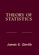Theory of Statistics (James E. Gentle)

This book is directed toward students for whom mathematical statistics is or will become an important part of their lives. It covers classical likelihood, Bayesian, and permutation inference; an introduction to basic asymptotic distribution theory; and modern topics.

•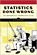Statistics Done Wrong: The Woefully Complete Guide (Reinhart)

Scientific progress depends on good research, and good research needs good statistics. But statistical analysis is tricky to get right, even for the best and brightest of us. You'd be surprised how many scientists are doing it wrong.

•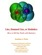Lies, Damned Lies: How to Tell the Truth with Statistics

The goal is to help you learn How to Tell the Truth with Statistics and, therefore, how to tell when others are telling the truth ... or are faking their "news". Covers Data Analysis, Binomial and normal models, Sample statistics, confidence intervals, hypothesis tests, etc.

•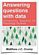Answering Questions with Data : Introductory Statistics

This is a free textbook teaching introductory statistics for undergraduates. Students will learn to select an appropriate data analysis technique, carry out the analysis, and draw appropriate conclusions.

Book Categories
 :All CategoriesTop Free BooksRecent BooksMiscellaneous BooksComputer EngineeringComputer LanguagesComputer ScienceData Science/DatabasesJava and Java EE (J2EE)Linux and UnixMathematicsMicrosoft and .NETMobile ComputingNetworking and CommunicationsSoftware EngineeringSpecial TopicsWeb Programming
Other Categories